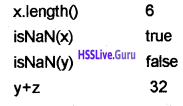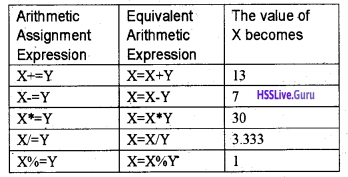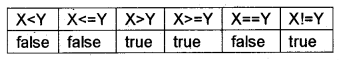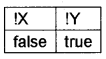# Plus Two Computer Science Chapter Wise Questions and Answers Chapter 6 Client-Side Scripting Using JavaScript

Students can Download Chapter 6 Client-Side Scripting Using JavaScript Questions and Answers, Plus Two Computer Science Chapter Wise Questions and Answers helps you to revise the complete Kerala State Syllabus and score more marks in your examinations

## Kerala Plus Two Computer Science Chapter Wise Questions and Answers Chapter 6 Client-Side Scripting Using JavaScript

### Plus Two Computer Science Client-Side Scripting Using JavaScript One Mark Questions and Answers

Question 1.
Among the following which one is the most correct. JavaScript is used mostly at the
(a) client side
(b) server side
(c) client side and server side
(a) client side

Question 2.
Name the tag that is used to embed scripts in a web page.
<SCRIPT>

Question 3.
In JavaScript, a variable is declared using the keyword
var

Question 4.
………………. are small programs embedded in the HTML pages.
ScriptsQuestion 5.
Who developed JavaScript?
Brendan Eich

Question 6.
………… are the scripts that are executed by the browser
Client Scripts

Question 7.
……………… are the scripts that are executed by the web server.
Server Scripts.

Question 8.
……………… Script is a platform independent script.
JavaScript

Question 9.
……………. Script is a platform dependent script.
VBScript

Question 10.
……………. makes the tags meaningful.
Attribute

Question 11.
……………. attribute specifies the name of the scripting language used.
Language

Question 12.
State True or False
The identifiers are case sensitive
True

Question 13.
Which part of the browser executes the JavaScript.
JavaScript engine

Question 14.
Odd one out
(b) Internet Explorer
(c) Mozilla Firefox
(d) C++
(d) C++, It is a programming language others are browsers.

Question 15.
A group of codes with a name is called …………
functionQuestion 16.
To declare a function the keyword ……………. is used.
function

Question 17.
A function contains a function …………. and function ………

Question 18.
State true or false
Even though a function is defined within the body section, it will not be executed, if it is not called.
True

Question 19.
Write down the purpose of the following code snippet
function print()
{
document.writefWelcome to JS”);
}
This code snippet is used to display the string, “Welcome to JS” on the screen (monitor)

Question 20.
From the following select which one is Not the data type in JavaScript
(a) Number
(b) String
(c) Boolean
(d) Time
(d) TimeQuestion 21.
…………… keyword is used to declare a variable in JavaScript.
var

Question 22.
…………. function is used to return the data type.
typeof()

Question 23.
……………….. is a special data type to represent variables that are not defined using var.
undefined

Question 24.
Odd one out
(a) +
(b) –
(c) %
(d) ==
(d) == , It is a relational operator the others are arithmetic operator

Question 25.
Odd one out
(a) &&
(b) ||
(c) !
(d) %
(d) %, it is an arithmetic operator, others are logical operator.

Question 26.
Odd one out
(a) <
(b) >
(c) ==
(d) !
(d) ! it is a logical operator, the others are relational operator.

Question 27.
Consider the following declaration
varb;
From the following which value can be used for the variable b as boolean value.
(a) True
(b) TRUE
(c) True
(d) FALSE
(a) True, The value is case sensitive

Question 28.
Predict the output of the following.
var a, b;
a = ”0480″;
b = 2828159;
document.write(a+b);
The output is “04802828159”. That is the string “0480″ concatenates(joins) the number 2828159. The output is a string, not a number.Question 29.
Predict the output
var x, y;
x = ”8″;
y = 3;
document. write(x+y);
The output is a string “83”; String addition means concatenation.

Question 30.
From the following which method is used to display a message (dialog box) on the screen.
(b) isNaN()
(c) toUpperCase()
(d) toLowerCase()

Question 31.
Raju wants to convert a lower case text to Upper case text, which function is to be used.
toUpperCase()

Question 32.
Christy wants to convert an upper case text to lower case text, which function is to be used.
tolower case()

Question 33.
Andrea wants to check a value is a number or not. From the following which function is used for that.
(a) isNumb()
(b) isNaN()
(c) isNotNumb()
(d) isNotNumber()
(b) isNaN()

Question 34.
Predict the output of the following code snippet
var x =”HIGHER SECONDARY”;
It displays a message box with character ‘E’ from the fifth (4 + 1) place

Question 35.
Read the following three statements regarding JavaScript.
(a) JavaScript can be used at the client side for data validation.
(b) JavaScript statements are case sensitive.
(c) JavaScript can be used only for creating web pages.
(d) All the three statements are correct
(d) All the three statements are correct

Question 36.
Write the output of the following web page.
<HTML>
<BODY>
<SCRIPT languge = “JavaScript”> document.write(“welcome”);
</SCRIPT>
welcome
</BODY>
</HTML>
welcome welcomeQuestion 37.
Following is the web page that accepts a string from a text box, converts in to upper case and display it on the screen. Complete the missing portion in the page.
<HTML>
<SCRIPT language = JavaScript”> function show()
{
var x, y;
x = ………….. ;
y = x.toupperCase ();
<SCRIPT>
<BODY>
<FORM name= “form1 ”>
Enter a string
<tNPUTtype=“text”name=“TEXTr>
<INPUT type= “submit” onClick() = “show()”> </FORM>
</BODY>
</HTML>
document.form1.text1.value

Question 38.
Name the attribute of <SCRIPT> tag that is used to include an external JavaScript file into a web page.
src

### Plus Two Computer Science Client-Side Scripting Using JavaScript Two Mark Questions and Answers

Question 1.
A javaScript code has the following three variables and values.
x = “Script”;
y = “3”;
z = “2″;
Then match the following table.Question 2.
“Placing JavaScript as an external file has some advantages”. Do you agree with this statement? Why?
External (another) JavaScript file:
We can write scripts in a file and save it as a separate file with the extension .js. The advantage is that this file can be used across multiple HTML files and can be enhance the speed of page loading.

Question 3.
Explain the difference between the statements.
document.write (“welcome”);
and
document.write () is a JavaScript command used to print anything on the browser window, document writes (“welcome”) prints “welcome” on the browser window. alert (“welcome”). This is a built in function used to display a message here the message “welcome” in a separate window.

Question 4.
Is it necessary to use Language – ‘JavaScript” in the <SCRIPT> tag to specify the JavaScript code? Why?
No, it is not necessary. If the language attribute is not specified, it will take the default value as Javascript.

Question 5.
Write the output of the following web page:
<HTML>
<BODY>
<SCRIPT language=”JavaScript”> function show ()
{
document.write(“welcome to JavaScript”);
}
</SCRIPT>
</BODY>
</HTML>
It will not display anything on the screen. This code snippet contains a function that will not do anything unless it is invoked(called).Question 6.
Write the output of the following web page:
<HTML>
<BODY>
<SCRIPT language -”JavaScript”>
function show ()
{
document.write (“welcome to JavaScript<br>”);
}
</SCRIPT>
show();
show();
<BODY>
</HTML>
The output is as follows
welcome to JavaScript
welcome to JavaScript
The message repeats 2 times.

Question 7.
Among the following, identify the data types used in JavaScript
int, float, number, char, boolean, long
From the list there is only two, number and boolean are the types used in JavaScript.

Question 8.
Write the output of the following web page and justify your answer.
<HTML>
<BODY>
<SCRIPT language =’’JavaScript”>
var x, y, z;
x =“10”;
y = “20”;
z = x+y;
document.write(z);
</SCRIPT>
</BODY>
</HTML>
x = “10” means x is a string variable y = “20” means y is a string variable x + y means the string x and y will be concatenated Hence it displays 1020

Question 9.
What do you mean by Scripts? Explain?
Scripts are small programs embedded in the HTML pages. <SCRIPT> tag is used to write scripts,
The attributes used are:

• Type – To specify the scripting language
• Src – Specify the source file

Two types of scripts:
1. Client scripts – These are scripts executed by the browser.
Eg: VB Script, Javascript, etc.

2. Server scripts – These are scripts executed by the server.
Eg: ASP, JSP, PHP, Perl, etc.

The languages that are used to write scripts are known as scripting languages.Question 10.
Suppose you have written a JavaScript function named checkData(). You want to execute the function when the mouse pointer is just moved over the button. How will you complete the following to do the same?
<INPUT Type=”button” ______ = “checkData()”>
<INPUT Type=”button” onMouseEnter = “checkData()”>

### Plus Two Computer Science Client-Side Scripting Using JavaScript Three Mark Questions and Answers

Question 1.
Categorize the following as UpperCamelCase or lowerCamelCase and explain.
(a) DateOfBirth
(b) dateOfJoining
(c) timeOfJoining
(d) PlaceOfBirth
CamelCase:
An identifier does not use special characters such as space hence a single word is formed using multiple words. Such a naming method is called CamelCase (without space between words and all the words first character is in upper case letter).

These are two types:
1. UpperCamelCase: when the first character of each word is capitalised,
2. lowerCamelCase: when the first character of each word except the first word is capitalised.

• UpperCamelCase: DateOfBirth, PlaceOfBirth
• lowerCamelCase: dateOfJoining, time Of Joining.

Question 2.
Explain the method of working of a JavaScript
Every browser has a JavaScript engine. If the code snippet contains JavaScript code, it is passed to the JavaScript engine for processing, the engine executes the code. If there is no script then it processes without the help of script engine. Hence an HTML file without JavaScript is faster than with JavaScript code.Question 3.
Write down the various data types used in JavaScript.

1. Number: Any number(whole or fractional) with or without a sign.
Eg: +1977,-38.0003,-100,3.14157,etc
2. String: It is a combination of characters enclosed within double-quotes.
Eg: “BVM”, “jobi_cg@rediffmail.com”, etc
3. Boolean: We can use either true or false.lt is case sensitive. That means can’t use TRUE OR FALSE

Question 4.
Explain how a variable is declaring in JavaScript
For storing values you have to declare a variable, for that the keyword var is used. There is no need to specify the data type.
Syntax:
var<variable name1> [, <variable name2>, <variable name3>,etc…]
Here square bracket indicates optional.
Eg: var x, y, z;
x =11;
y = “BVM”;
z = false;
Here x is of number type, y is of string and z is of Boolean type.

Question 5.
What are the different ways to add Scripts to a web page?
The three different ways to add Scripts as follows
1. Inside <BODY> section
Scripts can be placed inside the <BODY> section.

Scripts can be placed inside the <HEAD> section. This method is widely accepted method

3. External (another) JavaScript file
We can write scripts in a file and save it as a separate file with the extension .js. The advantage is that this file can be used across multiple HTML files and can be enhance the speed of page loading.

Question 6.
Create a web page that checks whether a student has passed or not?
<HTML>
<title>
JavaScript-Passed or not </title>
<SCRIPTLanguage=”JavaScript”> function check()
{
var score;
score=document.frmCheck.txtscore.value; . if(score<30)
alert(“The student is failed”); else ;
}
</SCRIPT>
<BODY>
<Form name=”frmCheck”>
Enter the score
<inputtype=”text” name=”txtscore”>
<br>
<input type=”button” value=”check” onClick= “check()”>
</Form>
</BODY>
</HTML>Question 7.
Create a web page to display the squares of first 10 numbers
<HTML>
<title>
JavaScript- squares
</title>
<BODY>
<SCRIPT Language=”JavaScript”> vari.s;
for(i=1; i<=10; i++)
{
s= i * i;
document.write(s);
document.write(“<br>”);
}
</SCRIPT>
</BODY>
</HTML>

Question 8.
Create a web page to display even numbers up to 10.
<HTML>
<title>
JavaScript- squares </title>
<BODY>
<SCRI PT Language=”JavaScript”>
vari;
i=2;
while(i<=10)
{
document.write(i); document.write(“<br>”); i +=2;
}
</SCRIPT>
</BODY>
</HTML>Question 9.
Following web page is used to show “Passed” or “Failed” based on a mark. Mark’less than 30 is cosidered as failed. There are some errors in the code. Correct them.
<HTML>
<BODY>
<SCRIPT src=”JavaScript”>
var m;
m = 55;
if(m<30)
document.print(“Passed”);
document.print(“Failed”);
<SCRIPT>
</BODY>
</HTML>
<HTML>
<BODY>
<SCRIPT language=”JavaScript”>
var m;
m = 55;
if(m<30)
document.write(“Passed”);
else
document.write(“Failed”);
</BODY>
</HTML>
</SCRIPT>

### Plus Two Computer Science Client-Side Scripting Using JavaScript Five Mark Questions and Answers

Question 1.
Consider the following declarations
vara, b, c, d;
a = “BVM”;
b = 100;
c = true;
d = 3.14157;
Predict the output of the following

1. document.write(typeof(a)); (1)
2. document.write(typeof(b)); (1)
3. document.write(typeof(c)); (1)
4. document.write(typeof(d)); (1)
5. document.write(typeof(e)); (1)

1. string
2. number
3. boolean
4. number
5. undefined

Question 2.
Create a web page to print the day of a week
<HTML>
<title>
JavaScript- day </title>
<SCRIPT Language=”JavaSCTipt”>
function checkData()
{
var n, ans;
n=Number(document.frmCheck.txtday. value); switch (n)
{
case 1;
ans=”Sunday”; break; case 2:
ans=”Monday”; break; case 3:
ans=”Tuesday”; break; case 4:
ans=”Wednesday”;
break;
case 5:
ans=”Thursday”;
break;
case 6:
ans=”Friday”;
break;
case 7:
ans=”Saturday”;
break;
default:
ans=”lnvalid”
}
}
</SCRIPT>
<BODY>
<Form name=”frmCheck”>
Enteranumber(1-7)
<inputtype=”text” name=”txtday”>
<br>
<input type=”button” va|ue=”Test” onClick= “checkData()”>
</Form>
</BODY>
</HTML>Question 3.
What is the operator? Explain in detail.
Operators are the symbols used to perform an operation
1. Arithmetic operators:
It is a binary operator. It is used to perform addition(+), subtraction(-), division (/), multiplication (*), modulus(%-gives the remainder), increment(++) and decrement(- -) operations.
Eg. If x = 10andy = 3thenlf x = 10 then
document.write(++x); → It prints 10 + 1 = 11 If x = 10 then
document.write(x++); → It prints 10 itself. If x = 10 then
document.write(—x); It prints 10 – 1 = 9 If x = 10 then
document.write(x—); → It prints 10 itself.

2. Assignment operators:
If a = 10 and b = 3 then a = b. This statement sets the value of a and b are same,i.e. it sets a to 3.
It is also called short hands lf X = 10 and Y = 3 then3. Relational(Comparision) operators:
It is used to perform comparison or relational operation between two values and returns either true or false.
Eg: if X =10 and Y = 3 then4. Logical operators:
Here AND(&&), OR(||) are binary operators and NOT (!) is a unary operator. It is used to combine relational operations and it gives either true or false If X = true and Y = false thenBoth operands must be true to get a true value in the case of AND(&&) operation. If X = true and Y = false thenEither one of the operands must be true to get true value in the case of OR(||) operation If X = true and Y = false thenThis is also called concatenation operator. joins(concatenates)two strings and forms a string.
Eg:
var x, y, z;
x = “BVM HSS;
y = “Kalparamba”;
z = x + y;
Here the variable z becomes “BVM HSS Kalparamba”.
Note: If both the operands are numbers then addition operator(+) produces number as a result otherwise it produces string as a result. Consider the followingQuestion 4.
Write down the control structures used in JavaScript.
Control structures in JavaScript, In general, the execution of the program is sequential, we can change the normal execution by using the control structures.
1. simple if Syntax:
if(test expression)
{
statements;
}
First, the test expression is evaluated, if it is true then the statement block will be executed otherwise not.
if-else Syntax:
if(test expression)
{
statement block1;
}
else
{
statement block2;
}
First, the test expression is evaluated, if it is true then the statement block1 will be executed otherwise statement block2 will be evaluated.
2. switch:
It is a multiple branch statement. Its syntax is given below, switch (expression)
{
case value1: statements;break;
case value2: statements;break;
case value3: statements;break;
case value4: statements;break;
case value5: statements;break;
default: statements;
}
First expression evaluated and selects the statements with matched case value.
Eg.
switch (n)
{
case 1: cout << “Sunday”;break;
case 2: cout << “Monday”;break;
case 3: cout << “Tuesday”;break;
case 4: cout << “Wednesday”;break;
case 5: cout << “Thursday”;break;
case 6: cout << “Friday”;break;
case 7: cout << “Saturday”;break;
default: cout << “lnvalid”
}
3. for loop
If a statement wants to execute more than once. Loop is used. for loop is an entry controlled loop.
The syntax of for loop is given below For(initialisation; testing; updation)
{
Body of the for loop;
}
while loop
If a statement wants to execute more than once Loop is used. It is also an entry controlled loop The syntax is given below
Loop variable initialised while(expression)
{
Body of the loop;
Update loop variable;
}
Here the loop variable must be initialised outside the while loop. Then the expression is evaluated if it is true then only the body of the loop will be executed and the loop variable must be updated inside the body. The body of the loop will be executed until the expression becomes false.Question 5.
Write down the different mouse events used in JavaScript.
Different mouse events and their description is given below

 Event Description onClick It occurs when the user clicks on an object by using mouse on mouse enter It occurs when the mouse pointer is moved onto an object on mouse leave It occurs when the mouse pointer is moved out of an object onKeyDown It occurs when the user presses a key on the keyboard on KeyUp It occurs when the user releases a key on the keyboard

Question 6.
Create a web page that displays the capital of a state.
<HTML>
<TITLE>
JavaScript </TITLE>
<SCRIPT Language=”JavaScript”> function capital()
{
varn.ans;
n=document.frmCapital.cbostate.selectedlndex; switch(n)
{
case 0:
ans=’Thiruvananthapuram”; i break;
case 1:
ans=”Bengaluru”;
break;
case 2:
ans=”Chennai”;
break;
case 3:
ans=”Mumbai”;
break;
}
document.frmCapital.txtCapital.value=ans;
}
</SCRIPT>
<BODY>
<FORM name=”frmCapital”>
<center>
State
<select size=1 name=”cbostate”>
<option>Kerala</option>
<option>Kamataka</option>